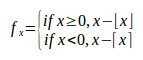### Writing a Multi-choice Math Formula in LibreOffice MathLibreOffice offers a Math editor which is very powerful and allows you to write complex Math Formulae. Unfortunately, it's not one to one compatible with LaTeX (in case you learned that Math Language) and so far I've not found pages that would explain all the different cases I often would like to use in my formulae.Get the book and learn everything that you need to know about LibreOffice Math. (Note: I'm an Amazon Affiliate.)

Indeed, a few things are not self explanatory (and even certain things have weird side effects, like using additional {} around sub-formulae when not required actually creates problems!?) and not readily available in their Elements Toolbar. The Multi-choice capability is one of them!

I wanted to write a formula where I have two choices. I want the fractional part of a number. The first case is when x is positive and the second when x is negative. Either case could be used for zero, although the formula I wrote uses positive or zero for that special case.

The final equation looks like this:In order to get the large brace bracket on the left side only, you use "left lbrace". The "lbrace" defines a left brace. The "left" asks for LibreOffice Math to resize it as required to fit whatever appears to the right side of the brace.

The right side needs to be an equivalent, but we do not want a brace on the right. This is actually very easy, we use "right none" instead of "right rbrace". This clearly means no right brace.

Now, within that formula, I wrote two conditions that needed to be separated by what I will call "an internal newline" (internal to the formula, at least.) This is accomplished by stacking. The "newline" command is used to separate formalae from each others. In other words, you can't use "newline" to separate two lines within the same formula like presented here. Instead, you stack them with the "stack" keyword and the "#" as the separator:

`stack { <?> # <?> }`

This will work with any number of lines. The syntax is very similar to a matrix except that it only needs to use a "#" instead of "##" to separate lines.

So now we have all the parts and the code of the final complete formula shown above looks like this:

```f_x =
left lbrace
stack {
if x >= 0, x - lfloor x rfloor #
if x < 0, x - lceil x rceil
}
right none```

In my case, both forumlae are about the same length, so I did not bother with any alignment. If yours are long and short, you will notice that by default it will center the choices, which looks weird. They should be left aligned. Only LibreOffice Math does not know that you are using the left brace for a choice so it fails in that way.

To change the alignment, you can use the "alignl" command as follow:

```f_x =
left lbrace
stack {
alignl if x >= 0, x - lfloor x rfloor #
alignl if x < 0, x - lceil x rceil
}
right none```

Now the two choices will be left aligned and the formula looks good.

Recent Posts on The Linux Page: